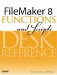# Let()

Category: Logical

 Syntax: Let ( {[} var1=expression1 {; var2=expression2 ...] }; calculation )

Parameters:

var(n)Any valid variable name. The rules for naming variables are the same as for defining fields.

expression(n)Any calculation formula, the results of which are assigned to the var(n) variable.

calculationAny calculation formula.

Parameters in curly braces { } are optional and may be repeated as needed, separated by a semicolon.

Data type returned: Text, Number, Date, Time, Timestamp, Container

Description:

The Let() function enables you to declare local variables within a calculation formula. The variables exist only within the boundaries of the Let() function itself.

The first parameter of the Let() function is a list of variable names and expressions. If multiple variables are declared, the list needs to be enclosed in square brackets. The items in the list are separated by semicolons. The variables are set in the order in which they appear. This means that you can use previously defined variables as part of the expression to define another variable.

The final parameter, calculation, is some expression that you want to evaluate. That formula can reference any of the variables declared in the first half of the function.

Duplicate variable names are allowed and variables can be named the same as existing fields. If this happens, the value assigned to the variable, not the field, will be used in future references to the variable within the Let() function.

Let() can be used to simplify complex, nested calculation formulas. We cannot advocate its use strongly enough.

In the case where a subexpression is used many times within a formula, the Let() function may also provide a performance benefit because the subexpression is evaluated only once when it is assigned to the variable.

You can also use the Let() function to set script variables like so:

``` Let ([
\$var = 100;
\$\$var = 500
];
expression
)
```

Keeping track of functions that overlap scopein this case overlapping the scope of a calculation expression with that of script or global variablescan often lead to code that is extrememly difficult to work with and maintain. While the preceding is entirely possible, we generally do not recommend it as a practice.

Note also that it is possible to use the Let() function to set a local script variable while no script is running. This in effect allows you to manipulate variables in a "null script" space that then do not exist while scripts run. A fairly obscure point, but interesting nonetheless.

Examples:

The following formula extracts the domain name from an email address:

``` Let([
start = Position (eMail ;"@" ;1 ;1);
numberOfCharacters = Length (eMail) - start];
Right ( eMail; numberOfCharacters)
)
```

The following example produces a summary of a students grades:

``` Let ([
CreditPoints = Sum (Classes::CreditPoints);
GPA = Round (TotalGradePoints/CreditPoints; 2)] ;
"Available Credit Points: " & CreditPoints & "¶" &
"Your GPA is: " & GPA
)
```

The final example formula returns the volume of a pyramid:

``` Let(
SideOfBase = 2 * Sqrt(2 * SlantHeight^2 - Height^2;
SideOfBase^2 * Height/3
)
```FileMaker 8 Functions and Scripts Desk Reference
ISBN: 0789735113
EAN: 2147483647
Year: 2004
Pages: 352

Similar book on Amazon